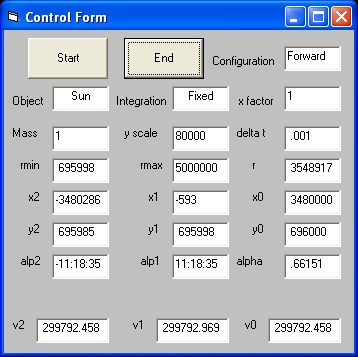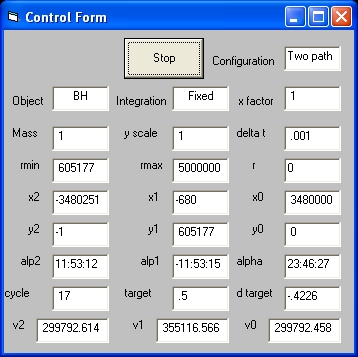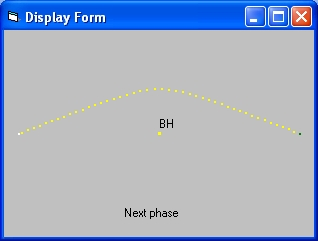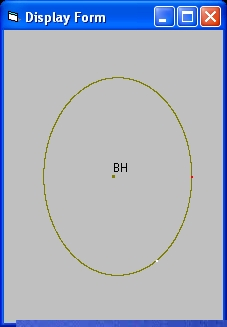## Visual Basic program "VB Light" - Description and operation

### Introduction and Purpose

The purpose of the Visual Basic program "VB Light" is to demonstrate how light is bended around the Sun using Newton's Law
A second more special phenomena is to demonstrate that the speed of light is not constant when gravitay is involved, also when using Newton's Law
This zip file contains 1 program:
• "VB Light.exe" written in Visual Basic.

The original program is written in QBASIC. For background information goto: Program "Light.bas": Light Bending around the Sun

### Description

The Visual basic program program "VB Light" consits of 2 Forms (or displays):
• Control Form. This form is used to Start the program and to modify the parameters.
• Display Form. This form shows the results of the simulation in a graphical form.

### Operation - Control Form

Operation of the program is done from the Control Form.Picture 1A - Forward Picture 1B - BackwardPicture 1C - Two path - phase 1 Picture 1D - Two path - final
Picture 1A Shows the Control Form after "Start" selection with configuration "Forward"
The Control Form shows 3 Commands: Start, End and Stop. This depends about were you are during program execution.
• The Start command is used to start the program
• The End command is used to terminate the program
• The Stop command is used to stop the simulation
After startup the Control Form shows 6 Control Parameters which can be modified:
Configuration,Object, dt calc , x factor , mass and y scale
All the other parameters are display only.
r,rmin, rmax , x0 , x1 , x2 , y0 , y1 , y2 v0 , v1 , v2 , alpha , alp1 , alp2 , cycle , target and d target

The following parameters can be modified:
• Configuration. This parameter has 3 values: "Forward", "Backward" and "Two path"
1. "Forward". In this case the simulation is only performed once. The Observer is "variable".
2. "Backward" In this case the simulation is performed many times. The Observer is "fixed".
3. "Two path". Same as "Backward" but with two sources.
• Object. This parameter has 4 values: "Sun", "Jupiter", "BH" and "Asymetric"
1 unit of mass of a BH is 10^5 Sun masses.
All the simulations are symmetric. That means the length of the trajectory approaching the object is the same as the length of the path towards the Observer.
With the value "Asymetric" this is not the case. The approaching trajectory is normally longer.
The parameter x factor is used to control this.
• dt calc This parameter has two values: "Fixed" or "Variable"
• x factor This parameter is used to control the length of the path approaching the object and the path towards the observer. In most cases this parameter influences both. When the object is "Asymetric" only the approaching path is affected.
• mass This parameter is preset after the object is selected. There after the parameter can be modified.
• y scale This parameter shows the amplification in the vertical or "y" direction

### Display Form

The "Display Form" shows the result of the simulation.

### "Configuration" = Forward

In order to simulate "Configuration = Forward" select:Object = "Sun" and Mass = 1
After this selection y scale changes to 80000 and rmax changes to 5000000
Next select "Start"Picture 2A Forward The display at the left shows the result of the simulation i.e. two lines: A "brown" line and a "yellow" line. The "brown" line represents the path of a lightray with a scale of 1. The position of the Center of the Sun is also drawn at that same scale, The "Yellow" line represents the path of a lightray with a y scale of 80000. This line shows that the path is bended. At a scale of 1 this is not visible. Both lines represents the same source. The source is at the same height or distance in vertical direction as the Radius of the Sun. That means because the distance is "short" the light ray passes close near the surface. In reality this is below the surface. You can test that because the parameter rmin = 695998 is smaller than the initial distance (the parameter Y0 = 696000) The angle alpha is = 0.85822 arcsec which is half the value predicted by General Relativity.
• Next change the parameter Mass = 2. The parameter y scale changes to 40000. Next select "Start".
• The angle alpha is = 1.716 which is close to the value predicted by General Relativity.

### "Configuration" = "Backward"

In order to simulate select:Object = "Asymetric" and Mass = 100
After this selection y scale changes to 800 and rmax changes to 4*5000000 = 20000000
Next change Configuration to "Backward" and select "Start"Picture 2B Backward The most important difference between "Backward" and "Forward" is, that in the "Backward" configuration the observer is at a "fixed" position and the chalenge of the simulation is to draw the path of a light ray starting from a "fixed" location towards this "fixed" Observer. As you can see from the simulation this goes in many tries. At each try the parameter cycle is increased. Both the "source" and the "Observer" are at the same height or distance in vertical direction At the end the simulation again shows two lines: A brown line and a yellow line. The brown line is drawn such that the "x scale" and the "y scale" are the same. In this particular situation the brown line is straight and does not show any bending The yellow line is drawn such that the vertical axis i.e. "y scale" is enlarged with the parameter "y scale" The yellow line shows that light is bended.
During the simulation the parameter y scale changes from 800 to 500
The final number of cycles is 7.

### "Configuration" = "Two Path"

The purpose of the "Two Path" configuration is to simulate lightbending from two different postions on the light source i.e a star.
• The first position is as if the center source star, the center of the Sun and the observer are all on one line.
• The second position is from the rim of the source star.
In order to simulate select:
• Object = "BH" and Mass = 1. The true mass of the BH in that case is 100000 Solar masses
After this selection y scale changes to 0.8 and rmax changes to 5000000
• Next change Configuration to "Two path"
• and select "Start"
• The display will show path 1. The display will be updated in roughly 17 cycles
• At the end the target Next phase will be displayed. Select this target.
• The display will show, besides path 1, also path 2.Picture 2C Two path - Initial with Next Picture 2D Two path - Final The bottom line shows the path of the light ray in the situation that the Light source, the Observer and the center of the BH are all on one straight line. That means from the point of view of the Observer the (center of the) Light source is behind the BH. The top line shows the path of the light ray of the rim of the source. This distance is equal to the Radius of the Sun. In this particular situation the simulation is symetric. That means you should also observe the same above as below the BH. To go one step futher: you should observe a ring around the BH.

### parameter "Integration" = "Fixed" and "Variable"

When parameter "Integration" is "Fixed" the step size or integration time dt is fixed and equal to the parameter "delta t"
When parameter "Integration" is "Variable" the step size or integration time dt is calculated as a function of the distance between the position of the photon and the position of the object i.e. the Sun
The same is done again when the photon is close to the observer.

In order to test the difference perform the following four simulations:
1. Set Configuration = "Forward", set Object = "Sun", set Integration = "Fixed". Set x factor = 100 and set mass = 2
The parameter "y scale" will become 400
Select Start. The display slowly builds up starting from the left.
After the simulation the parameter "delta t"=0.001. This is the standard value.
The parameter alpha = 1.75043
2. Keep everything the same but change Integration into "Variable".
Select Start. The display quickly builds up starting from the left.
After the simulation the parameter "delta t"=0.01679. This is the average value.
The parameter alpha = 1.75043
3. Change x factor = 1000. The parameter "y scale" will become 40
Select Start. The display quickly builds up starting from the left.
After the simulation the parameter "delta t"=0.12594. This is the average value.
The parameter alpha = 1.75043
4. Change x factor = 10000. The parameter "y scale" will become 4
Select Start. The display quickly builds up starting from the left.
After the simulation the parameter "delta t"=1.00756.This is the average value.
The parameter alpha = 1.75043

### The speed of light

What the simulations clearly show that the speed of light when a light ray passes an object is not constant. The higest speed is at the closest distance near the rim of the object i.e. Sun, Jupiter, or BH.
In order to demonstrate this phenomena the parameters: v0 , v1 and v2 are used.
• The parameter v0 shows the speed of light at the source. This is the same value as the speed of light in vacuum.
• The parameter v1 shows the speed of light at the shortest distance of the object being studied.
• The parameter v2 shows the speed of light near the observer (mass less).
The results of different tests are shown in the Displays: 1A, 1B, 1C and 1D

The following table shows the values (v1) of the speed of light at the nearest approach.
 Object Configuration Integration Mass v1 v2 Cycle Sun Forward Fixed 1 299792.969 299792.458 Sun Forward Fixed 100 299843.595 299792.459 Sun Forward Fixed 1000 300304.412 299792.557 Sun Backward Fixed 1 299792.969 299792.458 8 Sun Backward Fixed 100 299843.528 299792.458 11 Sun Backward Fixed 1000 300297.917 299792.458 11 Jupiter Forward Fixed 0.000954 299792.462 299792.458 BH Two path Fixed 1 355116.566 299792.614 17 BH Two path Fixed 1 339919.761 300039.478 17
What the table shows are small increases in speed of light (v1) for Sun sized values and large values for BH's

### The parameter alp2

 ``` . . . . . . alp2 alp1 . O-----------X-----------S Observer object Source ```
The parameter alpha 1 is the angle near the source between the path of the lightray from the source and the straight line between the source and observer
The parameter alpha 2 is the angle near the observer between the path of the lightray towards the observer and the the straight line between the observer and source
Together the two parameters alpha 1 and alpha 2 define the parameter alpha. This is the total reflection angle.

Consider the following experiments: (Only phase 1 is performed)
 # object mass Integration Configuration x factor rmin x0 alp1 alp2 alpha cycle 1 Asymetric 100000 Variable Two path 10 1298538 34800000 -2:21:39 9:21:55 11:43:34 23 2 Asymetric 200000 Variable Two path 20 2597085 69600000 -2:21:39 9:21:54 11:43:34 23 3 Asymetric 250000 Variable Two path 25 3246352 87000000 -2:21:39 9:21:55 11:43:34 24 4 Asymetric 100000 Variable Two path 20 1888928 69600000 -1:40:09 6:38:57 8:19:06 18 5 Asymetric 125000 Variable Two path 25 2361163 87000000 -1:40:09 6:38:56 8:19:06 15 6 BH 1 Variable Two path 10 2132505 34800000 -3:44:05 3:44:05 7:28:11 20 7 BH 2 Variable Two path 20 4264976 69600000 -3:44:05 3:44:05 7:28:11 20 8 BH 4 Variable Two path 10 4041720 34800000 -7:29:09 7:29:09 14:58:18 16
What is important in this table that in certain cases the angle "alpha 2" is identical.
What that means is that these situations the observer sees a ring in the sky, with the same radius, but that the mass of the object that caused the ring is different.

Consider the following experiments:
 # object mass Integration Configuration x factor alp1 alp2 cycle 1 BH 1 Variable Two path 100 -1:10:49 1:10:49 19 2 BH 2 Variable Two path 200 -1:10:49 1:10:49 21 3 Asymetric 100000 Variable Two path 640 -0:17:42 1:10:48 37+ 4 Asymetric 130000 Variable Two path 832 -0:17:42 1:10:48 29

### Reflection 1

Page 1101 of the book "Gravitation" by MTW in paragraph "40.3 Light deflection" shows Figure 40.2 which is redrawn as Figure 40.2.1 at the left side below.
 ``` ..........>.......A . . Star B . . * S * . * * x Earth * * . -------------* Sun * . * * . * * * * ``` ```..>..A . . C . Star . B . * S . * * x Earth * * . -------------* Sun * . * * . * * * * ``` Figure 40.2.1 Figure 40.2.2
The above two pictures are rotated 90 degrees counter clockwise as it image Figure 40.2
Figure 40.2.1 represents the picture from the book "Gravitation"
The left side of each figure represents the source of the light path i.e. a star at "infinite" distance
The problem with Figure 40.2.1 (Figure 40.2) is that the shortest distance of the light ray is perpendicular above the Sun, at the point marked S, which coincides with the point A of the light path. Starting from point A the light path is bended until point B. This is wrong. The light path should have been drawn symmetrical around the shortest distance.
This problem is corrected in Figure 40.2.2. In that figure the light path is symmetrical around the point C. That means the line segments A-C and c-B are of the same length and the line S-C represents the shortest distance.

It is strange that this figure is wrong, because it raises the question what is wrong in the text.

### Reflection 2 - BH

Perform the following 4 experiments
In each cas Select "Start" and roughly 10 seconds later select "Stop", because the simulation does not stop by it self
 # Configuration Object mass Integration x factor radius 1 Forward BH -5 Fixed 1 696000 2 Forward BH -50 Fixed 10 6960000 3 Forward BH -500 Fixed 100 69600000 4 Forward BH -4713 Variable 1000 696000000
Picture 3 shows the result of each simulation.
What the display shows is a photon which revolves around the BH. In principle you can have a circular disc (a cloud of photons) which rotates around the BH. This photons are invisible for an observer outside the BH.
The red dot represents the initial position of the photon. The "white" dot represents the position when the simulation is stopped.Picture 3 BH

### Feedback

None

Created 21 January 2016